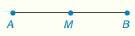Chapter 1.CR, Problem 22CR### Elementary Geometry for College St...

6th Edition
Daniel C. Alexander + 1 other
ISBN: 9781285195698

#### Solutions

Chapter
Section### Elementary Geometry for College St...

6th Edition
Daniel C. Alexander + 1 other
ISBN: 9781285195698
Textbook Problem
1 views

# Given: A M = 3 x − 1 M B = 4 x − 5 M is the midpoint of A B ¯ Find: A BTo determine

To find:

The value of AB.

Explanation

Given:

AM=3x1

MB=4x5

And,

M is the midpoint of AB¯.

The Figure (1) is given as,

Figure (1)

Concept:

The midpoint of a line is that separates the given line into two equal lengths or two equal segments.

Calculation:

Since, M is the midpoint of AB¯, then it can be written as,

AM=MB(1)

AB=2AM(2)

Substitute 3x1 for AM and 4x5 for MB in the above equation (1).

### Still sussing out bartleby?

Check out a sample textbook solution.

See a sample solution

#### The Solution to Your Study Problems

Bartleby provides explanations to thousands of textbook problems written by our experts, many with advanced degrees!

Get Started

#### In Exercises 7-10, solve for x or y. 7+y2=3

Calculus: An Applied Approach (MindTap Course List)

#### True or False: The figure at the right is the graph of the direction field for y = (y 1)x2.

Study Guide for Stewart's Single Variable Calculus: Early Transcendentals, 8th

#### j × (−k) = i −i j + k −j − k

Study Guide for Stewart's Multivariable Calculus, 8th

#### Distinguish between science and pseudoscience.

Research Methods for the Behavioral Sciences (MindTap Course List)